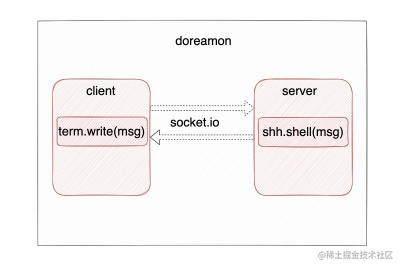# 如何搭建一个简易的 Web Terminal（一）

### 前言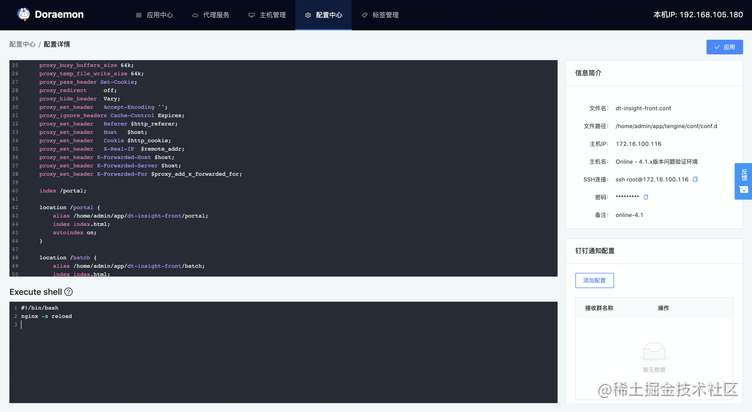### Xterm.js

Xterm.js 是一个用 TypeScript 编写的前端组件，它可以让应用程序在浏览器中为用户带来功能齐全的终端。它被 VS Code、Hyper 和 Theia 等流行项目使用。

#### 基本 API

• Terminal

``````import { Terminal } from 'xterm';

const term = new Terminal();

• onKey、onData

Terminal 实例上监听输入事件的函数

• write

Terminal 实例上写入文本的方法

Terminal 实例上加载插件的方法

• attach 、fit 插件

fit 插件可以适配调整 Terminal 的大小，使得其适配 Terminal 的父元素

attach 插件提供了将终端附加到 WebSocket 流的方法，以下是官网使用的例子

``````import { Terminal } from 'xterm';

const term = new Terminal();
const socket = new WebSocket('wss://docker.example.com/containers/mycontainerid/attach/ws');

// Attach the socket to term

#### 基本使用

1、首先第一步是安装 Xterm

npm install xterm / yarn add xterm

2、使用 xterm 生成 Terminal 实例对象，将其挂载到 dom 元素上

``````// webTerminal.tsx
import React, { useEffect, useState } from 'react'
import { Terminal } from 'xterm'

import './style.scss';
import 'xterm/css/xterm.css'

const WebTerminal: React.FC = () => {
const [terminal, setTerminal] = useState(null)

const initTerminal = () => {
const prefix = 'admin \$ '
const terminal: any = new Terminal({ cursorBlink: true })

terminal.open(document.getElementById('terminal-container'))
// terminal 的尺寸与父元素匹配

terminal.writeln('\x1b[1;1;32mwellcom to web terminal!\x1b[0m')
terminal.write(prefix)
setTerminal(terminal)
}

useEffect(() => { initTerminal() }, [])

return (
<div id="terminal-container" className='c-webTerminal__container'></div>
)
}
export default WebTerminal

``````// style.scss
.c-webTerminal__container {
width: 600px;
height: 350px;
}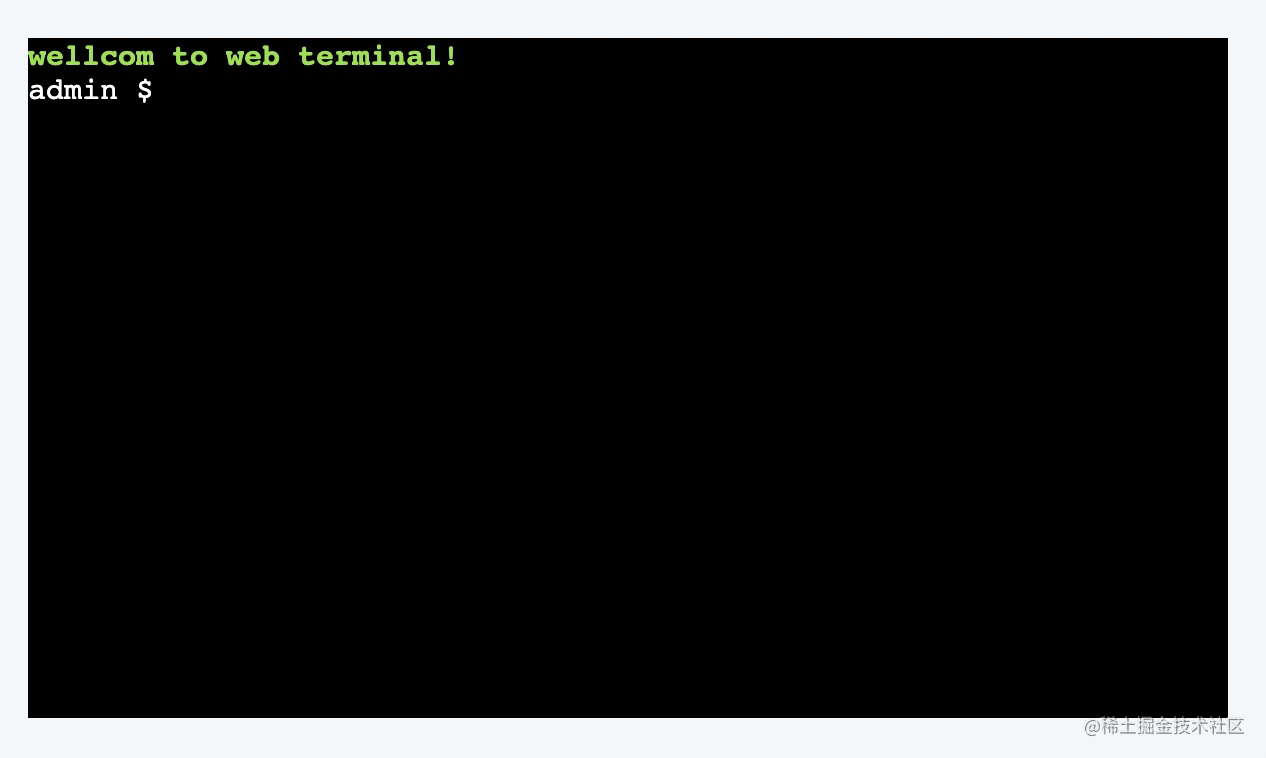#### 输入操作

##### 基本输入

``````// webTerminal.tsx
...
const WebTerminal: React.FC = () => {
const [terminal, setTerminal] = useState(null)
const prefix = 'admin \$ '

let inputText = '' // 输入字符

const onKeyAction = () => {
terminal.onKey(e => {
const { key, domEvent } = e
const { keyCode, altKey, altGraphKey, ctrlKey, metaKey } = domEvent

const printAble = !(altKey || altGraphKey || ctrlKey || metaKey) // 禁止相关按键
const totalOffsetLength = inputText.length + prefix.length   // 总偏移量
const currentOffsetLength = terminal._core.buffer.x     // 当前x偏移量

switch(keyCode) {
...
default:
if (!printAble) break
if (totalOffsetLength >= terminal.cols)  break
if (currentOffsetLength >= totalOffsetLength) {
terminal.write(key)
inputText += key
break
}
const cursorOffSetLength = getCursorOffsetLength(totalOffsetLength - currentOffsetLength, '\x1b[D')
terminal.write('\x1b[?K' + `\${key}\${inputText.slice(currentOffsetLength - prefix.length)}`) // 在当前的坐标写上 key 和坐标后面的字符
terminal.write(cursorOffSetLength)  // 移动停留在当前位置的光标
inputText = inputText.slice(0, currentOffsetLength) + key + inputText.slice(totalOffsetLength - currentOffsetLength)
}
})
}

useEffect(() => {
if (terminal) {
onKeyAction()
}
}, [terminal])

...
...
}

// const.ts
export const TERMINAL_INPUT_KEY = {
BACK: 8, // 退格删除键
ENTER: 13, // 回车键
UP: 38, // 方向盘上键
DOWN: 40, // 方向盘键
LEFT: 37, // 方向盘左键
RIGHT: 39 // 方向盘右键
}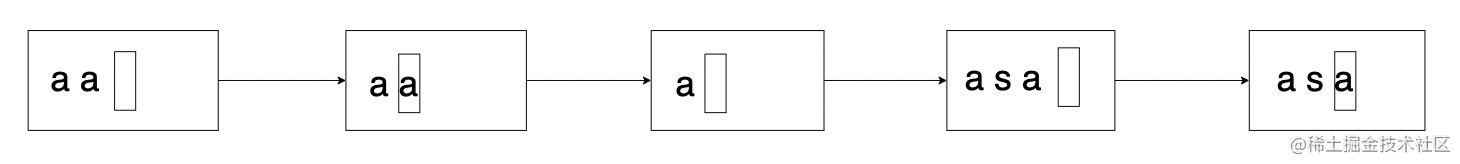1、光标移到第二位，按下键盘输入字符 s

2、删除光标位置到字符末尾的字符

3、将输入的字符与原有字符文本的光标位置到行末的字符拼接写入

4、将光标移到原有的输入位置

##### 删除操作
``````// webTerminal.tsx
...
const getCursorOffsetLength = (offsetLength: number, subString: string = '') => {
let cursorOffsetLength = ''
for (let offset = 0; offset < offsetLength; offset++) {
cursorOffsetLength += subString
}
return cursorOffsetLength
}

...
case TERMINAL_INPUT_KEY.BACK:
if (currentOffsetLength > prefix.length) {
const cursorOffSetLength = getCursorOffsetLength(totalOffsetLength - currentOffsetLength, '\x1b[D') // 保留原来光标位置

terminal._core.buffer.x = currentOffsetLength - 1
terminal.write('\x1b[?K' + inputText.slice(currentOffsetLength-prefix.length))
terminal.write(cursorOffSetLength)
inputText = `\${inputText.slice(0, currentOffsetLength - prefix.length - 1)}\${inputText.slice(currentOffsetLength - prefix.length)}`
}
break
...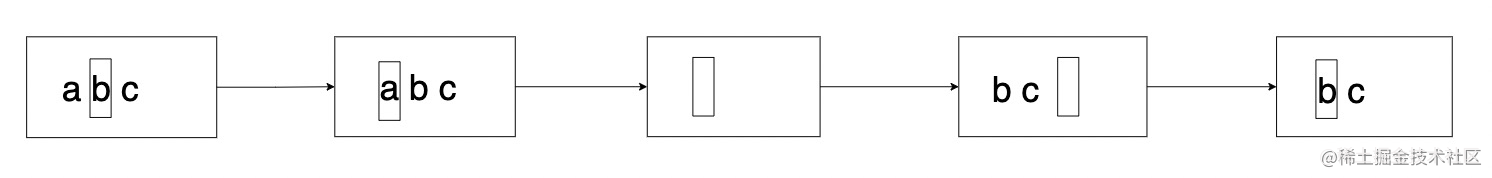1、光标移到第二位，按下键盘删除字符

2、清除当前的光标位置到末尾的字符

3、根据偏移量拼接剩余字符

3、将光标移到原有的输入位置

##### 回车操作
``````// webTerminal.tsx
...
let inputText = ''
let currentIndex = 0
let inputTextList = []

const handleInputText = () => {
terminal.write('\r\n')
if (!inputText.trim()) {
terminal.prompt()
return
}

if (inputTextList.indexOf(inputText) === -1) {
inputTextList.push(inputText)
currentIndex = inputTextList.length
}

terminal.prompt()
}

...
case TERMINAL_INPUT_KEY.ENTER:
handleInputText()
inputText = ''
break
...

##### 向上/向下操作
``````// webTerminal.tsx
...
case TERMINAL_INPUT_KEY.UP: {
if (!inputTextList[currentIndex - 1]) break

const offsetLength = getCursorOffsetLength(inputText.length, '\x1b[D')

inputText = inputTextList[currentIndex - 1]
terminal.write(offsetLength + '\x1b[?K' )
terminal.write(inputTextList[currentIndex - 1])
terminal._core.buffer.x = totalOffsetLength
currentIndex--

break
}
...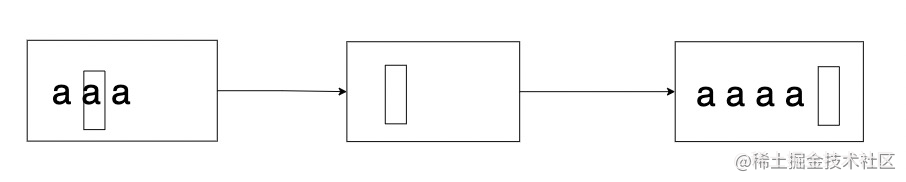##### 向左/向右操作
``````// webTerminal.tsx
...
case TERMINAL_INPUT_KEY.LEFT:
if (currentOffsetLength > prefix.length) {
terminal.write(key) // '\x1b[D'
}
break

case TERMINAL_INPUT_KEY.RIGHT:
if (currentOffsetLength < totalOffsetLength) {
terminal.write(key) // '\x1b[C'
}
break
...

#### 待完善的点

1、接入 websocket，实现服务端和客户端之间的通信

2、接入 ssh，目前只是添加了终端的输入操作，我们最终的目的还是需要让它能够登陆到服务器上面